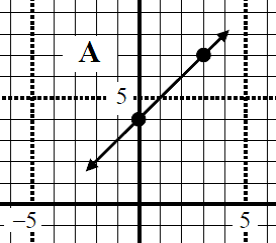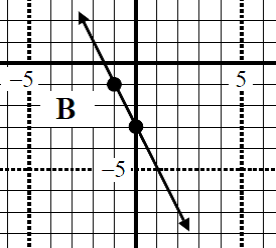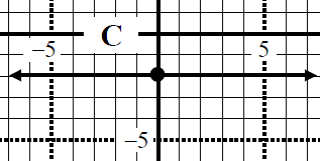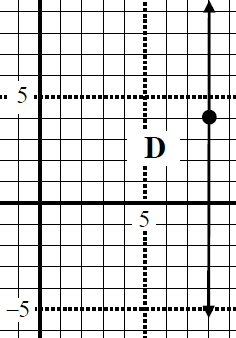# Parallel line equation

### Parallel line equation

In this lesson, we will look at questions related to parallel line equation. We will try to determine parallel line equation with different given information such as, graphs, equations of other lines and points.

#### Lessons

• 1.
Given the graph of linear equation, find the slope of parallel line equation.
a)b)c)d)• 2.
The lines 2y - 6x - 4 = 0 and 6y - cx = 0 are parallel. Find "c".

• 3.
Determine the equation of a line that is parallel to the line y = 4x - 1, and passes through the point (2, - 4). Answer in slope intercept form and general form.

• 4.
Determine the equation of a line that is parallel to the line 3y - 9x - 3 = 0, and has the same x-int as the line 5y - 9x + 20 = 0. Answer in slope intercept form and general form.